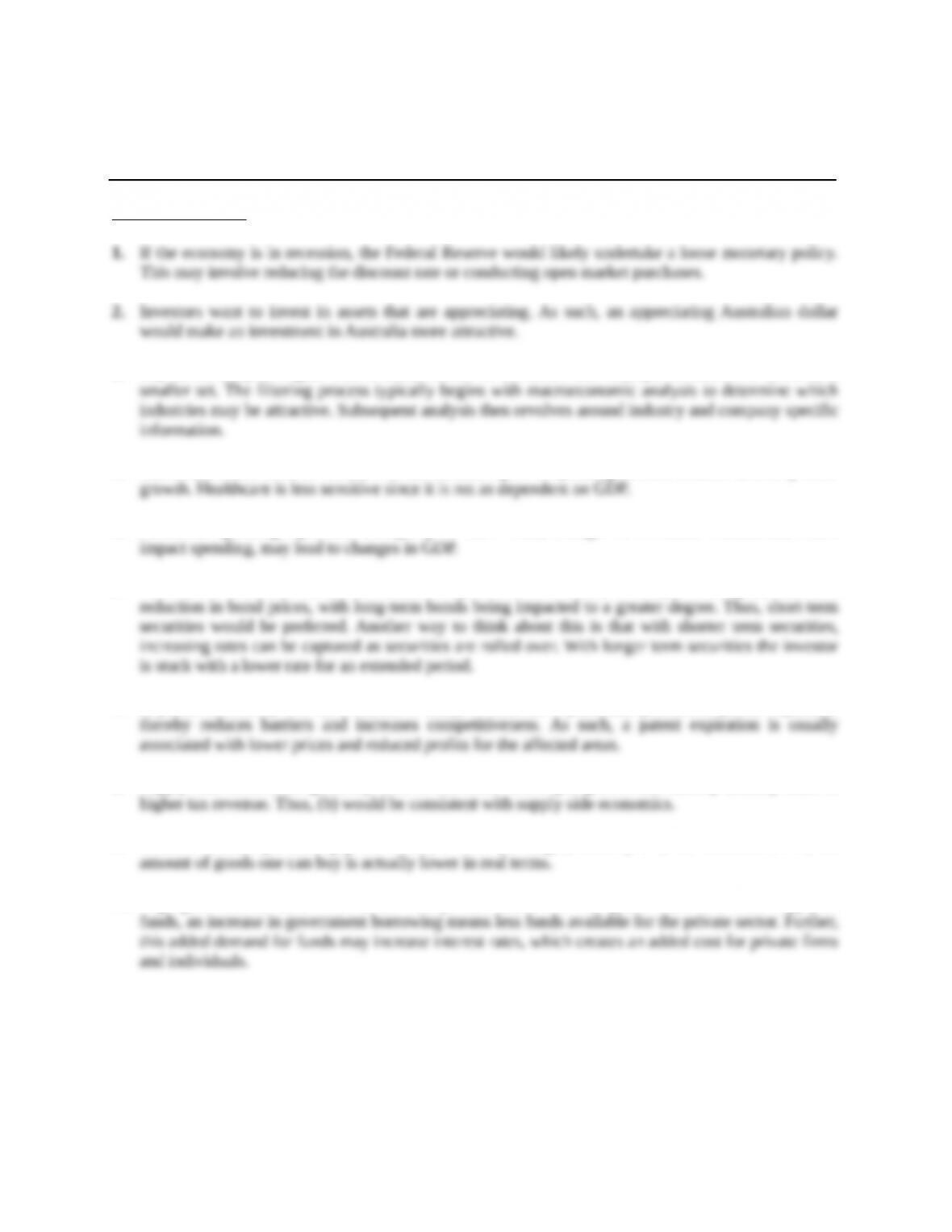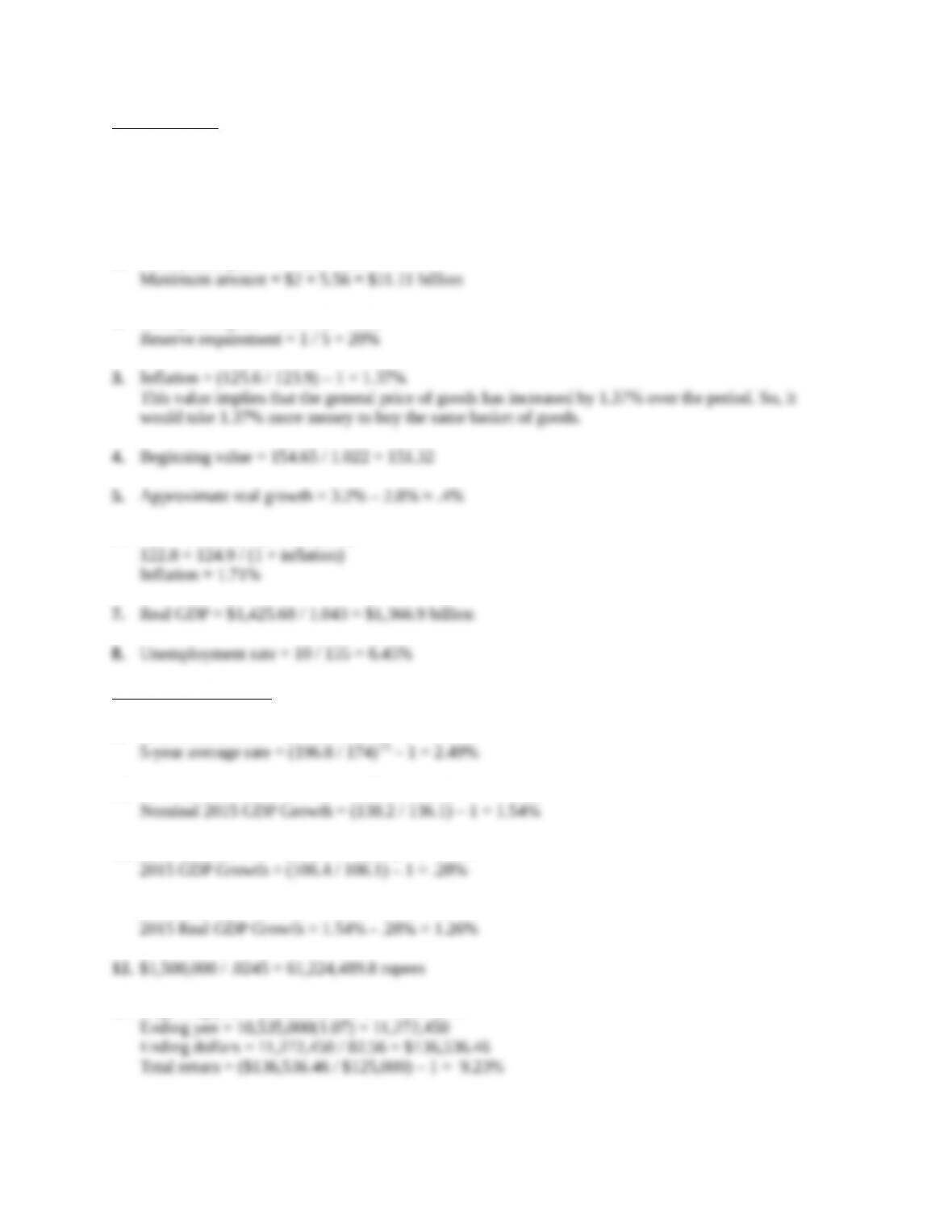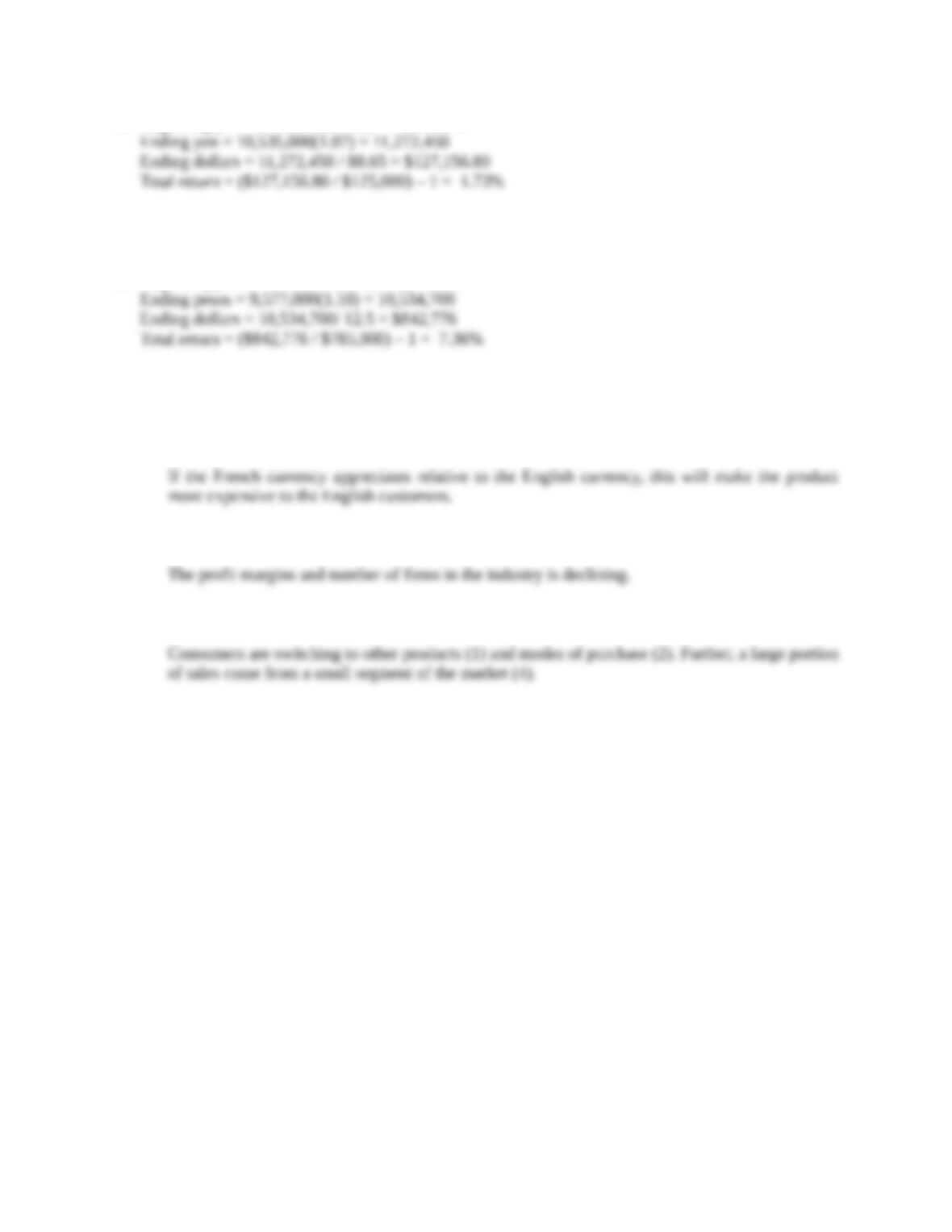Type
Solution Manual
Book Title
Fundamentals of Investments: Valuation and Management 8th Edition
ISBN 13
978-1259720697

### 978-1259720697 Chapter 19 Solution Manual

January 2, 2020Chapter 19
Global Economic Activity and Industry Analysis
Concept Questions
3. Top-down analysis begins with a broad choice of securities and attempts to narrow it down to a
4. Industrials would be more sensitive to the business cycle as spending on such items is driven by GDP
5. Consumer spending represents a majority of GDP. Thus, changes in consumer confidence, which
6. If inflation increases, interest rates would likely follow. An increase in rates, all else equal, leads to a
7. A patent effectively acts as a barrier to entry, at least for a particular product. A patent expiration
8. Supply-side economists generally believe that lower taxes spur growth and thereby actually leads to
9. Inflation reduces purchasing power. Thus, if GDP (or wages) rises by 3%, but inflation is 5%, the
10. High governmental debt “crowds out” the private sector. For example, if there is a fixed supply of
Education.Core Questions
NOTE: All end of chapter problems were solved using a spreadsheet. Many problems require multiple
steps. Due to space and readability constraints, when these intermediate steps are included in this
solutions manual, rounding may appear to have occurred. However, the final answer for each problem is
found without rounding during any step in the problem.
1. Money multiplier = 1 / .18 = 5.56
2. Money multiplier = 300 / 60 = 5.00
6. Real = nominal / (1 + inflation)
Intermediate Questions
9. 2015 Inflation rate = (196.8 / 190.3) – 1 = 3.42%
10. Nominal 2014 GDP Growth = (136.1 / 125.4) – 1 = 8.53%
11. 2014 Inflation rate = (106.1.1 / 105.3) – 1 =.76%
2014 Real GDP Growth = 8.53% - .76% = 7.77%
13. Beginning yen = \$125,000(84.28) = 10,535,000
Education.14. Beginning yen = \$125,000(84.28) = 10,535,000
The total return comes from the investment return and the exchange rate movement. In this case, the
yen depreciated, thereby offsetting some of the investment return that was earned.
15. Beginning pesos = \$785,000(12.2) = 9,577,000
CFA Exam Review by Kaplan Schweser
1. a
2. a
3. c
4. b